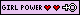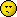# Well..

General — Page 
SolidKAYOS2007 Jun 24 • 84
68 ₧
phoenix_r said:
no love from teh havokk?!
16:39:40 EDT
phoenix_r said:
dont make me put you on my list, right under fedex_

Ya i just now found this. I dont check the chat that often. and at the time i was busy.

<3 Happy?
Make awkward sexual advances, not war.
Down Rodeo said:
Dammit, this was the one place that didn't have this, but noooooo, molkman pisses all over that≡ 2009 May 20 at 16:58 PDTjrkookid
Half Irish, half NOT FUNNY2007 May 27 • 1415
110 ₧
I rest my case: Foolnix_Rapist is retarded.
 ≡ 2009 May 20 at 17:18 PDTthe_cloud_system
polly pushy pants2008 Aug 1 • 3076
-6 ₧
lol this thread is detacated to him
I drink to forget but I always remember.
 ≡ 2009 May 20 at 20:05 PDTphoenix_r2009 May 13 • 902
17 ₧
<3<3<3<3<3<3<3<3<3<3<3<3<3<3
<3<3<3<3<3<3<3<3<3<3<3<3<3<3
<3<3<3<3<3<3<3
<3<3<3<3<3<3<3
<3<3<3<3<3<3<3<3<3<3<3<3<3<3
<3<3<3<3<3<3<3
<3<3<3<3<3<3<3
<3<3<3<3<3<3<3<3<3<3<3<3<3<3
<3<3<3<3<3<3<3
<3<3<3<3<3<3<3
<3<3<3<3<3<3<3<3<3<3<3<3<3<3
BOO
 ≡ 2009 May 21 at 11:17 PDT — Ed. 2009 May 21 at 11:21 PDTPage